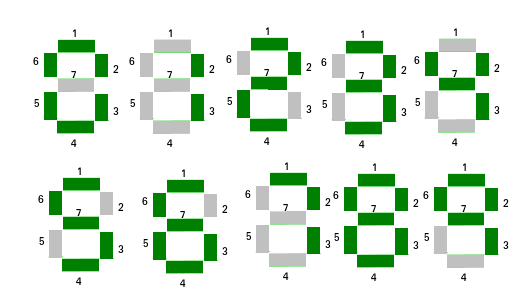GeeksforGeeks App
Open AppBrowser
Continue

# Check if mirror image of a number is same if displayed in seven segment display

Given a positive number n. The task is to check if the mirror image of the number is equal to given number or not if displayed on Seven Line Segment. A mirror image of a number is a reflected duplication of the number that appears almost identical but is reversed in the direction perpendicular to the mirror surface.
Examples:

```Input : n = 101
Output: Yes
Mirror image of 101 is 101 on seven line segment. So, print "Yes".

Input : n = 020
Output : No```Observe each digit when displayed on seven line segment, only digit 0, 1, 8 remain same in their mirror image. So, for a number to be equal to its mirror image, it should contain only 0, 1, 8 digits. Also, observe, for two number to be equal their corresponding position digit should be same. Therefore, mirror image should also contain the same digit on their corresponding digit position. So, the number should also be a palindrome.
Below is implementation of this approach:

## C++

 `// C++ Program to check if mirror image of a number is``// same if displayed in seven segment display``#include ``using` `namespace` `std;` `// Return "Yes", if the mirror image of number``// is same as the given number``// Else return "No"``string checkEqual(string S)``{``    ``// Checking if the number contain only 0, 1, 8.``    ``for` `(``int` `i = 0; i < S.size(); i++) {``        ``if` `(S[i] != ``'1'` `&& S[i] != ``'0'` `&& S[i] != ``'8'``) {``            ``return` `"No"``;``        ``}``    ``}` `    ``int` `start = 0, end = S.size() - 1;` `    ``// Checking if the number is palindrome or not.``    ``while` `(start < end) {` `        ``// If corresponding index is not equal.``        ``if` `(S[start] != S[end]) {``            ``return` `"No"``;``        ``}` `        ``start++;``        ``end--;``    ``}` `    ``return` `"Yes"``;``}``int` `main()``{``    ``string S = ``"101"``;` `    ``cout << checkEqual(S) << endl;``    ``return` `0;``}`

## Java

 `// Java Program to check if``// mirror image of a number``// is same if displayed in``// seven segment display``import` `java.io.*;` `class` `GFG``{``    ` `// Return "Yes", if the``// mirror image of number``// is same as the given``// number Else return "No"``static` `String checkEqual(String S)``{``    ``// Checking if the number``    ``// contain only 0, 1, 8.``    ``for` `(``int` `i = ``0``;``             ``i < S.length(); i++)``    ``{``        ``if` `(S.charAt(i) != ``'1'` `&&``            ``S.charAt(i) != ``'0'` `&&``            ``S.charAt(i) != ``'8'``)``        ``{``            ``return` `"No"``;``        ``}``    ``}` `    ``int` `start = ``0``,``        ``end = S.length() - ``1``;` `    ``// Checking if the number``    ``// is palindrome or not.``    ``while` `(start < end)``    ``{` `        ``// If corresponding``        ``// index is not equal.``        ``if` `(S.charAt(start) !=``            ``S.charAt(end))``        ``{``            ``return` `"No"``;``        ``}` `        ``start++;``        ``end--;``    ``}` `    ``return` `"Yes"``;``}` `// Driver Code``public` `static` `void` `main (String[] args)``{``    ``String S = ``"101"``;` `    ``System.out.println(checkEqual(S));``}``}` `// This code is contributed``// by anuj_67.`

## Python3

 `# Python3 Program to check if mirror``# image of a number is same if displayed``# in seven segment display` `# Return "Yes", if the mirror image``# of number is same as the given number``# Else return "No"``def` `checkEqual(S):``    ` `    ``# Checking if the number contain``    ``# only 0, 1, 8.``    ``for` `i ``in` `range``(``len``(S)):``        ``if` `(S[i] !``=` `'1'` `and` `S[i] !``=` `'0'``                        ``and` `S[i] !``=` `'8'``):``            ``return` `"No"``;` `    ``start ``=` `0``;``    ``end ``=` `len``(S) ``-` `1``;` `    ``# Checking if the number is``    ``# palindrome or not.``    ``while` `(start < end):` `        ``# If corresponding index is not equal.``        ``if` `(S[start] !``=` `S[end]):``            ``return` `"No"``;` `        ``start ``+``=` `1``;``        ``end ``-``=` `1``;` `    ``return` `"Yes"``;` `# Driver Code``S ``=` `"101"``;``print``(checkEqual(S));``    ` `# This code is contributed by mits`

## C#

 `// C# Program to check if mirror image``// of a number is same if displayed in``// seven segment display``using` `System;` `class` `GFG``{``    ` `// Return "Yes", if the mirror image``// of number is same as the given``// number Else return "No"``static` `string` `checkEqual(``string` `S)``{``    ``// Checking if the number``    ``// contain only 0, 1, 8.``    ``for` `(``int` `i = 0; i < S.Length; i++)``    ``{``        ``if` `(S[i] != ``'1'` `&&``            ``S[i] != ``'0'` `&&``            ``S[i] != ``'8'``)``        ``{``            ``return` `"No"``;``        ``}``    ``}` `    ``int` `start = 0, end = S.Length - 1;` `    ``// Checking if the number is``    ``// palindrome or not.``    ``while` `(start < end)``    ``{` `        ``// If corresponding index is not equal.``        ``if` `(S[start] !=``            ``S[end])``        ``{``            ``return` `"No"``;``        ``}` `        ``start++;``        ``end--;``    ``}` `    ``return` `"Yes"``;``}` `// Driver Code``public` `static` `void` `Main()``{``    ``string` `S = ``"101"``;` `    ``Console.WriteLine(checkEqual(S));``}``}` `// This code is contributed``// by mits`

## PHP

 ``

## Javascript

 ``

Output

`Yes`

Time Complexity: O(n), where n is the size of the given string
Auxiliary Space: O(1), as no extra space is required

My Personal Notes arrow_drop_up